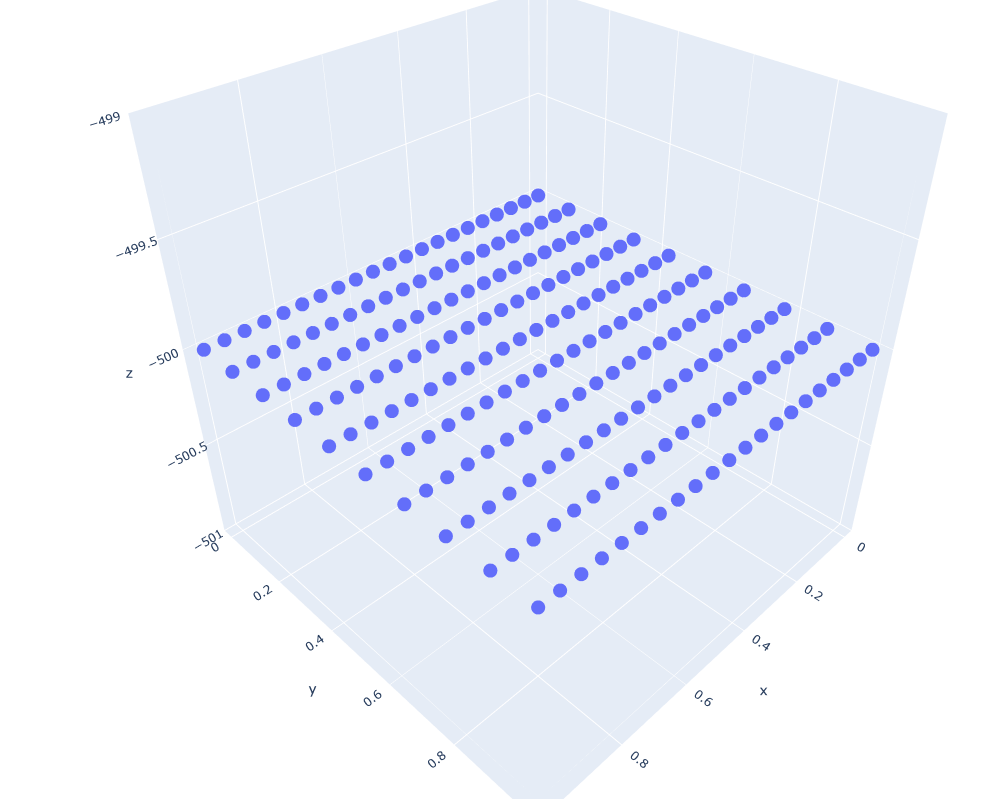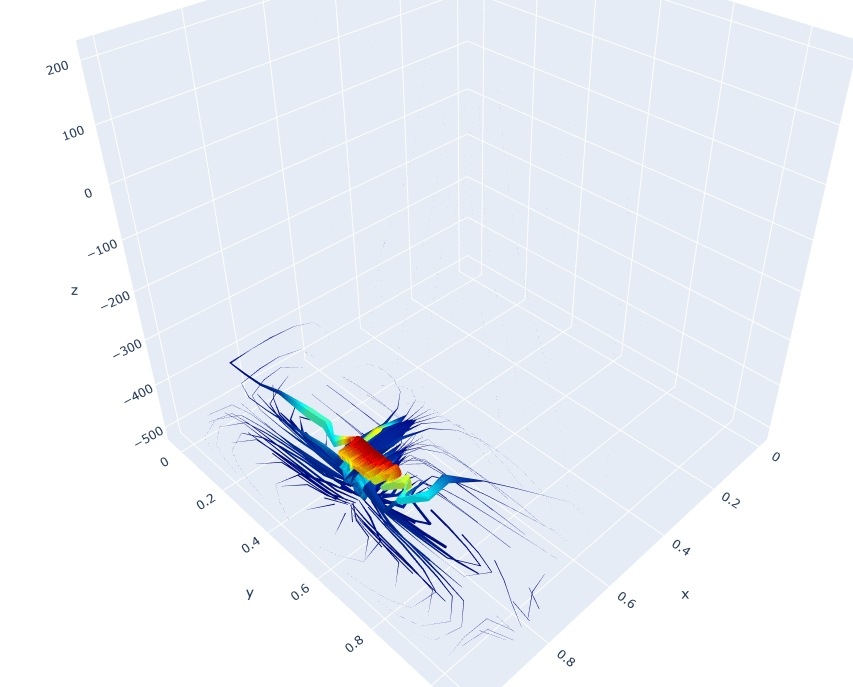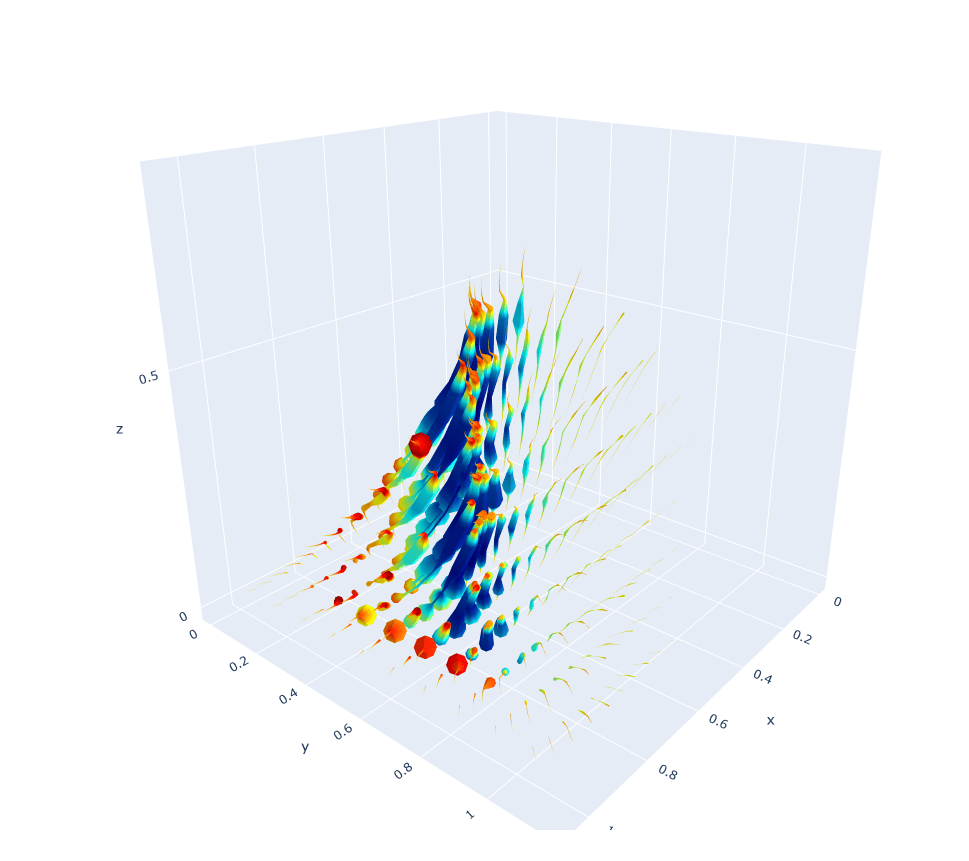# Aim

I would like to create a 3D Streamtube Plot with Plotly.

Here is a cross-section of the vector field in the middle of the plot to give you an idea of how it looks like:The final vector field should have rotational symmetry.

# My Attempt

2. Run the code bellow:

Code:

``````import plotly.graph_objs as go
import plotly.express as px
import pandas as pd
import numpy as np

import plotly.io as pio
pio.renderers.default='browser'

# Import data to pandas
# Plot

X = np.linspace(0,1,101)
Y = np.linspace(0,1,10)
Z = np.linspace(0,1,101)

# Points from which the streamtubes should originate
xpos,ypos = np.meshgrid(X[::5],Y, indexing="xy")
xpos = xpos.reshape(1,-1)
ypos = ypos.reshape(1,-1)

starting_points = px.scatter_3d(
x=xpos,
y=ypos,
z=[-500]*len(xpos)
)
starting_points.show()

# Streamtube Plot

data_plot = [go.Streamtube(
x = df['x'],
y = df['y'],
z = df['z'],
u = df['u'],
v = df['v'],
w = df['w'],
starts = dict(                           #Determines the streamtubes starting position.
x=xpos,
y=ypos,
z=[-500]*len(xpos)
),
#sizeref = 0.3,
colorscale = 'jet',
showscale = True,
maxdisplayed = 300                      #Determines the maximum segments displayed in a streamtube.
)]

fig = go.Figure(data=data_plot)
fig.show()
``````

The initial points (starting points) of the streamtubes seem to be nicely defined:…but the resulting 3D streamtube plot is very weird:Edit:

I tried normalizing the field plot, but the result is still not satisfactory:

``````import plotly.graph_objs as go
import pandas as pd
import numpy as np

import plotly.io as pio
pio.renderers.default='browser'

# Import data to pandas

# NORMALIZE VECTOR FIELD -> between [0,1]
df["u"] = (df["u"]-df["u"].min()) / (df["u"].max()-df["u"].min())
df["v"] = (df["v"]-df["v"].min()) / (df["v"].max()-df["v"].min())
df["w"] = (df["w"]-df["w"].min()) / (df["w"].max()-df["w"].min())

# Plot

X = np.linspace(0,1,101)
Y = np.linspace(0,1,10)
Z = np.linspace(0,1,101)

# Points from which the streamtubes should originate
xpos,ypos = np.meshgrid(X[::5],Y, indexing="xy")
xpos = xpos.reshape(1,-1)
ypos = ypos.reshape(1,-1)

# Streamtube Plot

data_plot = [go.Streamtube(
x = df['x'],
y = df['y'],
z = df['z'],
u = df['u'],
v = df['v'],
w = df['w'],
starts = dict(                           #Determines the streamtubes starting position.
x=xpos,
y=ypos,
z=*len(xpos)
),
#sizeref = 0.3,
colorscale = 'jet',
showscale = True,
maxdisplayed = 300                      #Determines the maximum segments displayed in a streamtube.
)]

fig = go.Figure(data=data_plot)
fig.show()
``````## Data

As for the data itself:

It is created from 10 slices (y-direction). For each slice (y), [u,v,w] on a regular xz mesh (101×101) was computed. The whole was then assembled into the dataframe which you can download, and which has 101x101x10 data points.

Get this bounty!!!

This site uses Akismet to reduce spam. Learn how your comment data is processed.